#DAY 3
0
Modern Web

## JavaScript 之旅 (3)：Exponentiation Operator (指數運算子)「JavaScript 之旅」系列文章發文於：

# 過去的 `Math.pow()`

``````let x = 2;

console.log(Math.pow(x, 2));  // 4

// 等同於
console.log(x * x);           // 4
``````

``````let x = 2;

console.log(Math.pow(x, 3));  // 8

// 等同於
console.log(x * x * x);       // 8
``````

``````let square = x => Math.pow(x, 2);
let cube = x => Math.pow(x, 3);

console.log(square(6));  // 36
console.log(cube(3));    // 27
``````

# 現代的 Exponentiation Operator

exponentiation 運算子是一種 infix notation (中綴表示法)，比用函數表示法還要更簡潔。

``````let square = x => x ** 2;
let cube = x => x ** 3;
``````

``````let a = 5;          // 00000000000000000000000000000101
let b = 3;          // 00000000000000000000000000000011

console.log(a ^ b); // 00000000000000000000000000000110
``````

``````console.log(NaN ** 2);  // NaN
console.log(2 ** NaN);  // NaN
``````

``````console.log(undefined ** 2);  // NaN
console.log(2 ** undefined);  // NaN
``````

``````console.log(null ** 2);  // 0
// 大約等同於 Number(null) ** 2，所以 0 ** 2 的計算結果為 0

console.log(2 ** null);  // 1
// 大約等同於 2 ** Number(null)，所以 2 ** 0 的計算結果為 1
``````

## Associativity

exponentiation 運算子的 associativity 是右到左 (即 right-associative)，也就是說，下面兩個寫法是相同的：

``````console.log(2 ** 3 ** 2);    // 512
console.log(2 ** (3 ** 2));  // 512

// 計算過程：
// 1. 2 ** 3 ** 2
// 2. 2 ** 9
// 3. 512
``````

``````console.log((2 ** 3) ** 2);  // 64

// 計算過程：
// 1. (2 ** 3) ** 2
// 2. 8 ** 2
// 3. 64
``````

• associative：可任意分組運算子
• left-associative：運算子從左側分組
• right-associative：運算子從右側分組
• non-associative：運算子不能 chained，通常是因為 output typ 和 input type 不相容

## Unary Operation 的用法

``````let a = 2;
let n = 3;

console.log(+a ** n);
console.log(-a ** n);
console.log(~a ** n);
console.log(!a ** n);
delete a ** n;
void a ** n;
typeof a ** n;

// SyntaxError: Unary operator used immediately before exponentiation expression. Parenthesis must be used to disambiguate operator precedence
``````

``````let a = 2;
let n = 3;

console.log(-2 ** 3);
console.log(-a ** n);
// SyntaxError: Unary operator used immediately before exponentiation expression. Parenthesis must be used to disambiguate operator precedence
``````

``````console.log((-2) ** 3);  // -8
``````

``````console.log(-(2 ** 10));  // -1024
``````

## Assignment Operator 的用法

exponentiation 運算子也有 assignment operator (賦值運算子)，語法是 `**=`

``````let x = 2;

x **= 3;
console.log(x);  // 8
``````

``````let x = 2;

x = x ** 3;
console.log(x);  // 8
``````

# Exponentiation Operator vs. `Math.pow()`

``````console.log(2n ** 3n);  // 8n

console.log(Math.pow(2n, 3n));  // TypeError: Cannot convert a BigInt value to a number
``````

# Sepc 定義

## Exponentiation Operator 的 spec 定義

exponentiation 運算子是 ECMAScript 內建 Numeric 型別 (Number 和 BigInt 型別都是 Numeric 型別) 的 operation：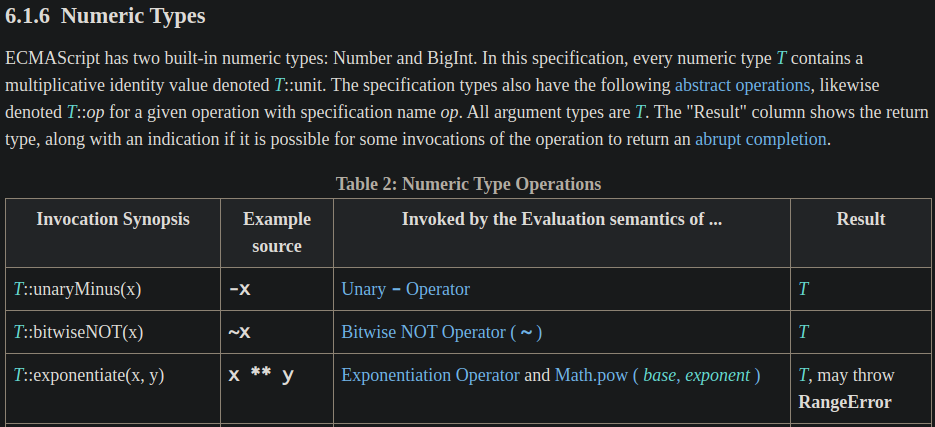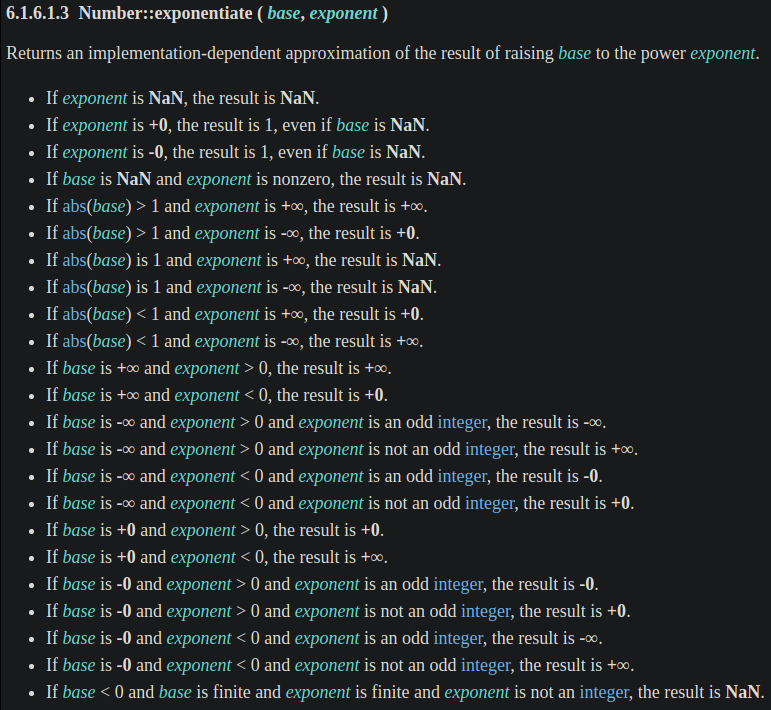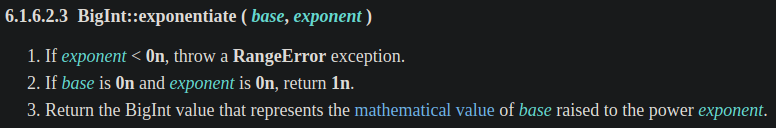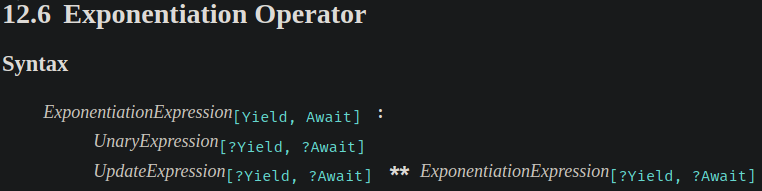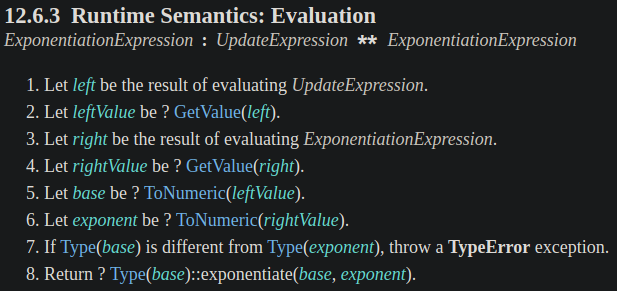``````console.log('2' ** '3');  // 8
``````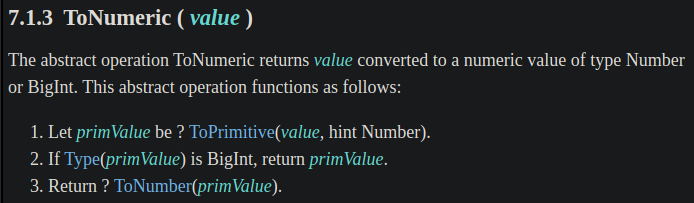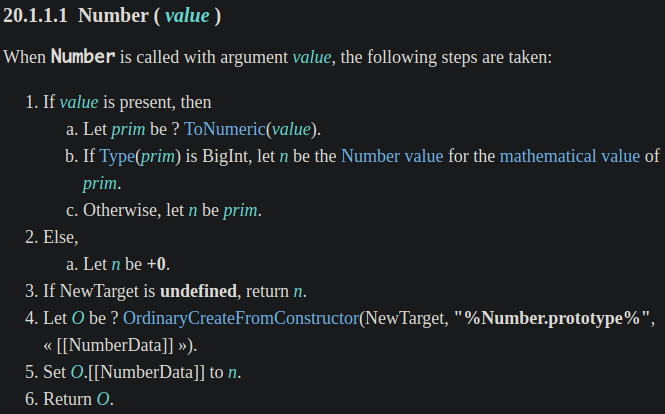`Number()` 才是我們真的可以使用的 constructor。

## `Math.pow()` 的 spec 定義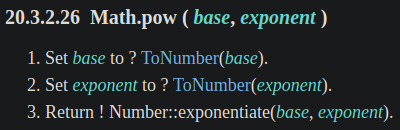# 資料來源

JavaScript 之旅30